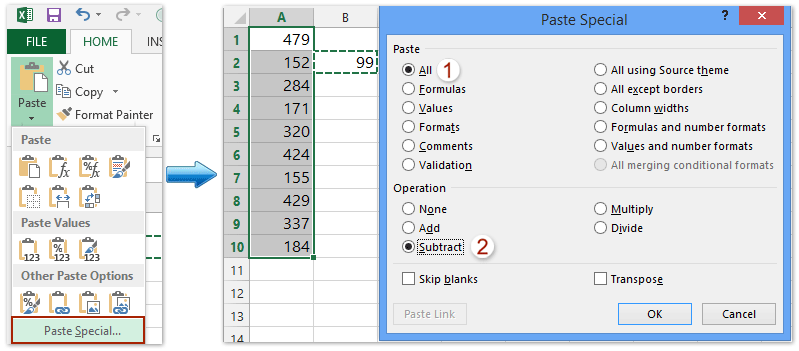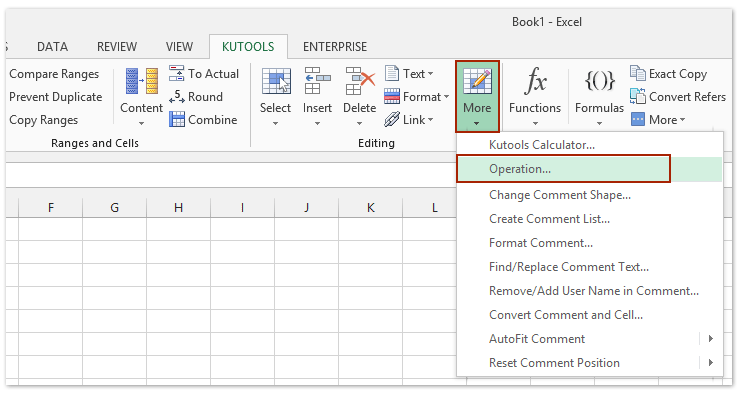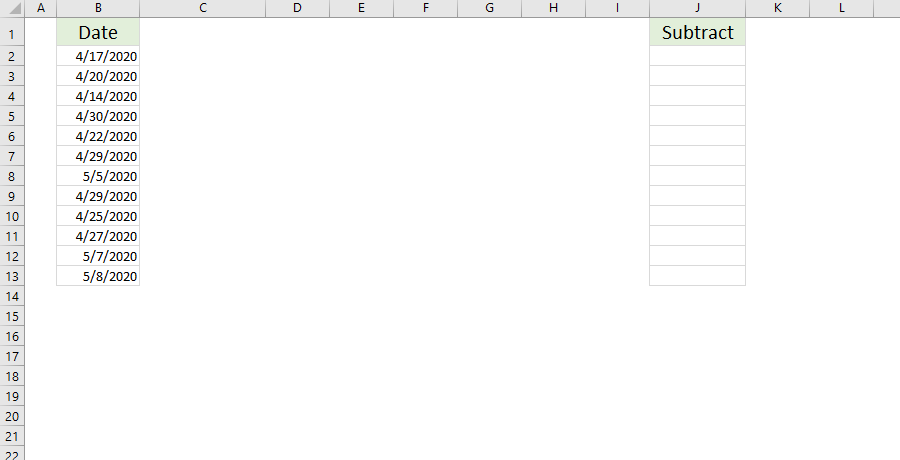Note: The other languages of the website are Google-translated. Back to English

## How to subtract a number from a range of cells in excel?

If you want to subtract a number such as 99 from a range of cells in Excel, how can you quickly subtract the number and get the calculated results?

#### Subtract a number from a range of cells with Paste Special function

With this method, you can do as the following steps:

1. Insert the number 99 into a blank cell and copy it.

2. Highlight the range that you would like to subtract the number from, and click Home > Paste > Paste Special. See screenshot below:3.In the Paste Special dialog box, select All option in the Paste section, check Subtract option in the Operation section, and click the OK button. See screenshot above:

The specified number 99 has been subtracted from the range of cells. And then delete the number 99.

#### Subtract a number from a range of cells with a Formula

Here a simple formula also can help you. Take the above data for example:

1. In cell C1, input the formula: =A1-99 (99 is a variable, it can be replaced by other numbers as you need), and press the Enter key.2. Keep the Cell C1 selected, and drag its AutoFill handle to the range as you need. And then all cells are extracted 99. See screenshot above.

3. As calculating results are formulas, you can copy these formulas cells, select a blank cell, right click and select the Values (V) to replace the formulas with their calculated results. See screenshot:#### Batch subtract a number from a range of cells without formula

With Kutools for Excel, you can not only apply the add, subtract, multiply, divide operation, but also can apply some complex calculation, such as rounding, exponentiation, custom expression or function operation.

Kutools for Excel - Includes more than 300 handy tools for Excel. Full feature free trial 30-day, no credit card required! Get It Now

If you have installed Kutools for Excel, please do as follows:

1．Select the range you want to subtract a number, and click Kutools > More > Operation Tools，see screenshot:2. In the Operation Tools dialog box, select Subtraction from Operation, input the number 99 in the Operand box, and then click OK or Apply.

And then the range of cells will be subtracted by the number 99. See screenshot:Note: If you want to create formulas as well, you can check Create formulas option. If the selected cells include formulas, and you don’t want to subtract the calculated results of formulas, please check Skip formula cells option.

Kutools for Excel - Includes more than 300 handy tools for Excel. Full feature free trial 30-day, no credit card required! Get It Now

#### Demo: subtract a number from a range of cells with/without formulas

Kutools for Excel includes more than 300 handy tools for Excel, free to try without limitation in 30 days. Download and Free Trial Now!

#### Easily add/subtract specific number of years, months, days, or weeks to dates in Excel

Kutools for Excel collects dozens of commonly used formulas to help Excel users quickly apply complex formula calculations without remembering them, such as add or subtract specific number of years/months/days/weeks to dates.       Full Feature Free Trial 30-day!.Apart from Add years/months/days/weeks to date formula and Add hours/minutes/seconds to date formula, the Commonly-used Formulas also contains Calculate age based on birthday formula, Sum absolute values formula, Count times a word appears formula, Sum numbers in a cell formula, etc.

Kutools for Excel - Includes more than 300 handy tools for Excel. Full feature free trial 30-day, no credit card required! Get It Now

### The Best Office Productivity Tools

#### Kutools for Excel Solves Most of Your Problems, and Increases Your Productivity by 80%

• Reuse: Quickly insert complex formulas, charts and anything that you have used before; Encrypt Cells with password; Create Mailing List and send emails...
• Super Formula Bar (easily edit multiple lines of text and formula); Reading Layout (easily read and edit large numbers of cells); Paste to Filtered Range...
• Merge Cells/Rows/Columns without losing Data; Split Cells Content; Combine Duplicate Rows/Columns... Prevent Duplicate Cells; Compare Ranges...
• Select Duplicate or Unique Rows; Select Blank Rows (all cells are empty); Super Find and Fuzzy Find in Many Workbooks; Random Select...
• Exact Copy Multiple Cells without changing formula reference; Auto Create References to Multiple Sheets; Insert Bullets, Check Boxes and more...
• Extract Text, Add Text, Remove by Position, Remove Space; Create and Print Paging Subtotals; Convert Between Cells Content and Comments...
• Super Filter (save and apply filter schemes to other sheets); Advanced Sort by month/week/day, frequency and more; Special Filter by bold, italic...
• Combine Workbooks and WorkSheets; Merge Tables based on key columns; Split Data into Multiple Sheets; Batch Convert xls, xlsx and PDF...
• More than 300 powerful features. Supports Office/Excel 2007-2019 and 365. Supports all languages. Easy deploying in your enterprise or organization. Full features 30-day free trial. 60-day money back guarantee.#### Office Tab Brings Tabbed interface to Office, and Make Your Work Much Easier

• Enable tabbed editing and reading in Word, Excel, PowerPoint, Publisher, Access, Visio and Project.
• Open and create multiple documents in new tabs of the same window, rather than in new windows.
• Increases your productivity by 50%, and reduces hundreds of mouse clicks for you every day!No ratings yet. Be the first to rate!
This comment was minimized by the moderator on the site
subratction through column
This comment was minimized by the moderator on the site
i want to Subtraction with formula like as use product witch use continue many number of multiply, sach as any formula of Subtraction.Sach as 1800 500 300 160 160 =680 Not use one by one to be continue.
This comment was minimized by the moderator on the site
I have a number like 500 and i have a tall list like , 20, 10, 50, 70, 90 and i will input in more data as when items are requested. i want a formula that will automatically subtract from the 500 any time a request is made. for example Mr. A requested 50 today so the difference now will be 450. if Mr. B also request 50 tomorrow i want excel to automatically deduct it from the stock of 500 and give me 400. Thanks.
This comment was minimized by the moderator on the site
I NEED TO FIND PERCENTAGE FOR A VALUE IN EXCEL EX:10000-2000=8000 2000=20% HOW SHOULD I APPLY THE FORMULA IN EXCEL PLS HELP ME
This comment was minimized by the moderator on the site
I want to find the remaining total. I have a number of units reported defective= 10 (column A). I have accepted 3 (column B) or I have scrapped 3 (column C) now I want to show the remaining quantity. How do I write the formula?
This comment was minimized by the moderator on the site
Hi,
If you need to make complex calculations for one or multiple numbers, you can apply Kutools for Excel’s Operation tool. In the operation Tools dialog box, specify the operation as Custom, and then type your formula in the Operand box.
This comment was minimized by the moderator on the site
I want to get the total by subtracting the number in the first column by the number in the second column, including both the numbers. Is there any formula?

I hope you could understand my question.

This comment was minimized by the moderator on the site
I have set up a Bank statement on my Excel program. I want to subtract the 1st total, add the 2nd total and that will equal my total.
This comment was minimized by the moderator on the site
How can I subtract two different random cells in a column depending on duplicate cells in another column
This comment was minimized by the moderator on the site
Hello, fawzy,